###### Descriptions and Examples for the POV-Ray Raytracerby Friedrich A. Lohmüller     Elementary Geometry for Raytracing
Italiano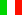Français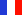Deutsch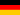Home
- POV-Ray Tutorial

- Geometrical Basics
for Raytracing

Right-angled Triangle
Pythagorean Theorem
Trigonometry Basics
Law of cosines
Equilateral Triangle
Regular Polygon
Polyhedron
Tetrahedron
Octahedron
Cube & Cuboid
Dodecahedron
Icosahedron
Cuboctahedron
Truncated Octahedron
Rhombicuboctahedron
Truncated Icosahedron
Circles
Tangent Circles
Internal Tangents
External Tangents

- Geometric 3D Animations

## External Tangents to two CirclesIn the following we write for the square root of a number the expression "sqrt(NUMBER)" and "abs(NUMBER)" for |NUMBER|, conforming to the syntax used in POV-Ray.Note: Here objects in 2D geometry are represented by 3D shapes in the xy-plane. Therefore all coordinates must have the z-components zero! ( <?,?,0>)

 We want the external tangents to the tangent points     T1 and T2 of two circles C1(M1,r1) and C2(M2,r2) with the radii r1 > r2 , as shown in the opposite image. The distance of their centers is d. The difference of their radii is ri = r1 - r2. The triangle M1,S,M2 has a right angle at S. The line(T1,T2) is parallel to the line(M2,S) and has the same length. So t = |T1,T2| = sqrt( d2 - ri2). The angle α = atan(ri/t). or   α = asin(ri/d). The calulation of the length of the belt around: The length of the segment around the circle C1: l1 = 2π·r1 ·(180+2·α)/360. The length of the segment around the circle C2: l2 = 2π·r2 ·(180-2·α)/360. The length of the complete belt is: l = l1 + l2 + 2·t .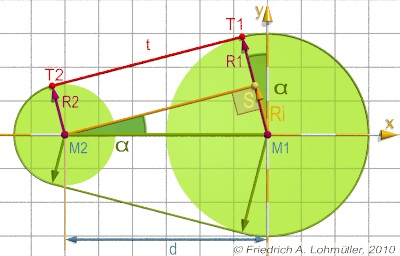External tangents to two circles rendered with POV-Ray

For what can we use this geometry?
Here some examples: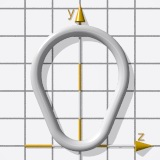A round conic torus.A round conic prism.A roller chain or a conveyor belt.
Animation tutorial:
'Roller Chain'A rolling bike chain.
Animation tutorial:
'Bike Chain'
See an animation here:
'Animation Bike Chain'
top
 © Friedrich A. Lohmüller, 2010 http://www.f-lohmueller.de